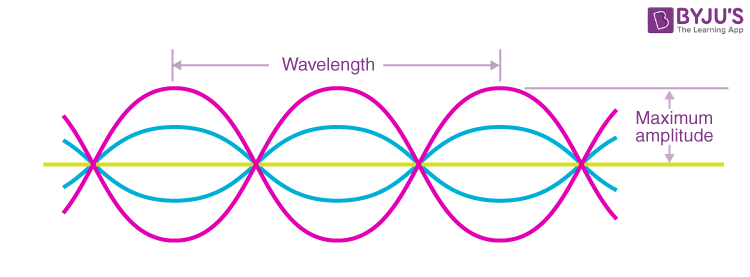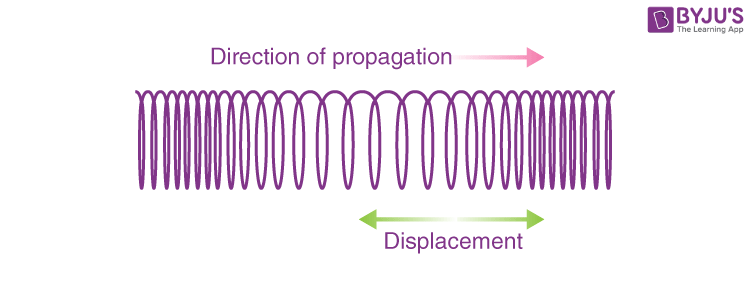# Transverse Waves and Longitudinal Waves

In Physics, waves are explained as an oscillation about the fixed point, accompanied by the transfer of energy travelling from one medium to another. When energy transfer occurs through a medium due to oscillation, the resultant wave can be termed a mechanical wave.

The medium of transmission limits the distance of the wave’s propagation. In this case, the oscillating material moves about a fixed point, and there is very little translational motion. One interesting property of mechanical waves is how they are measured, given by displacement divided by wavelength. When it reaches 1, this dimensionless factor results in the generation of harmonic effects. For example, waves break on the beach when this factor exceeds one, resulting in turbulence.

## Types of Mechanical Waves

There are primarily two types of mechanical waves, namely:

• Transverse Waves
• Longitudinal Waves

## What Are Transverse Waves?

In transverse waves, the displacement of the particle is perpendicular to the direction of propagation of the wave. Below is the picture which shows the structure of transverse waves.### Examples Of Transverse Waves

In transverse waves, too, the particles do not move along with the wave. They move up and down about their equilibrium positions. Some examples of transverse waves are:

• The ripples on the surface of the water
• The secondary waves of an earthquake
• Electromagnetic waves
• The waves on a string
• The ocean waves

 Gravity Waves Gravity waves are also known as gravitational waves and are defined as the ripples caused in space. It is caused due to various reasons like two huge stars orbiting each other or the explosion of stars asymmetrically. Sound Waves – Medium for their Propagation Sound waves are mechanical waves that are defined as the motion of waves with compression (high-pressure region) and rarefaction (low-pressure region).

## What are Longitudinal Waves

In a longitudinal wave, the displacement of the particle is parallel to the direction of the wave propagation. What you see in the picture is the wavefront progressing forward and the particles compressing and expanding in the same direction. This wave is marked by periodic compression and rarefaction zones, where the medium expands.

### Examples of Longitudinal Waves

The particles in the wave do not move along with the wave; they simply oscillate back and forth about their own equilibrium.

• Sound waves in air
• The primary waves of an earthquake
• Ultrasound
• The vibration of a spring
• The fluctuations in a gas
• The tsunami waves### Examples of Transverse and Longitudinal Waves

We have learned that the P waves (also known as Primary waves) are examples of longitudinal waves while the S waves (also known as Secondary waves) are examples of transverse waves.

Further, we shall learn about the examples that combine longitudinal and transverse waves.

• Water waves:
Water waves are an example of both longitudinal and transverse waves. The movement of particles in water waves is in a clockwise direction. While the movement of the waves is in a transverse manner. We also need to understand that the radius of the particles decreases with an increase in the depth of the water.
• Rayleigh surface waves:
Rayleigh surface waves are another example of a combination of both longitudinal and transverse waves. These waves involve both up and down motion and side-to-side motion. Rayleigh wave is also known as the seismic wave, which causes the shaking of the earth in an elliptical motion. Of all the known seismic waves, the Rayleigh waves can produce a long wave duration on the seismographs.

## Video lecture on longitudinal and transverse waves## Frequently Asked Questions – FAQs

Q1

### State whether the given statement is true or false: Waves are the transfer of momentum and energy via a medium.

The above-given statement is true.
The propagation of waves takes place only through a medium. So, it is right to say that there is a transfer of energy and momentum from one particle to another during the propagation of the waves.
Q2

### State whether the given statement is true or false: For the propagation of mechanical waves, there is no requirement for a medium.

The above-given statement is false.
Mechanical waves require or are dependent on the medium for their propagation. This is because the particles of these waves need a medium to propagate. Their transfer of energy takes place only through a medium. This is why sound waves do not travel in a vacuum, as there are no particles to transport the energy.
Q3

### State whether the given statement is true or false: The polarisation phenomenon is possible in sound waves.

The above-given statement is false.
For the phenomenon of polarisation to occur, the wave’s particles need to be perpendicular to the direction of propagation. Since sound waves are longitudinal, this phenomenon cannot be executed by sound waves. This is possible in light waves.
Q4

### What is node in wave?

A node for a standing wave is defined as the point at which the amplitude is zero. For a standing wave, the wave is said to be stable at the node.
Also, a standing wave is defined as a wave in a medium with every point associated with a constant amplitude. The amplitude of this wave is zero at the nodes while maximum at the antinodes.
Q5

### What are the characteristics of transverse waves?

The following are the characteristics of transverse wave:
• The propagation of transverse waves is possible only through solids and not through liquids or gases.
• Only transverse waves can exhibit the phenomenon of polarisation. The vibration of the particles in a medium takes place in the same place; this is known as the plane of vibration or polarisation.
• Properties such as pressure and density are constant in a medium when transverse waves are propagated.
• The formation of typical crests and troughs in transverse waves is periodic in nature.
• The propagation of transverse waves depends on the medium’s rigidity.
Q6

### What is a mechanical wave?

A mechanical wave is a type of wave produced when energy transfer occurs through a medium due to oscillation.
Q7

### What are the types of mechanical waves?

Types of mechanical waves are:

• Transverse Waves
• Longitudinal Waves
• Q8

### Give an example of longitudinal waves

The vibration in spring is one of the ideal examples of longitudinal waves.

Q9

### Electromagnetic wave is a longitudinal wave or a transverse wave?

An electromagnetic wave is a transverse wave.
Q10

### Give an example of the combination of both longitudinal and transverse waves.

Rayleigh surface waves are an example of transverse and longitudinal waves.